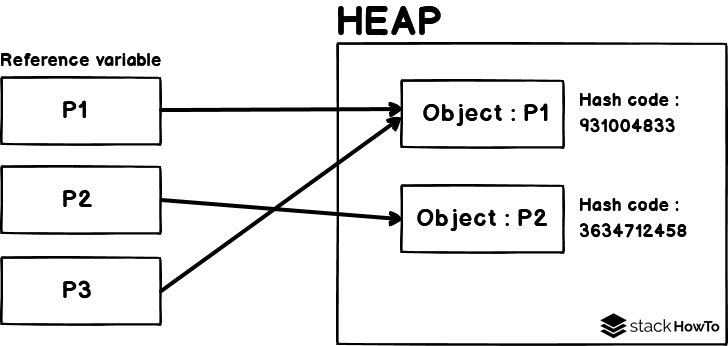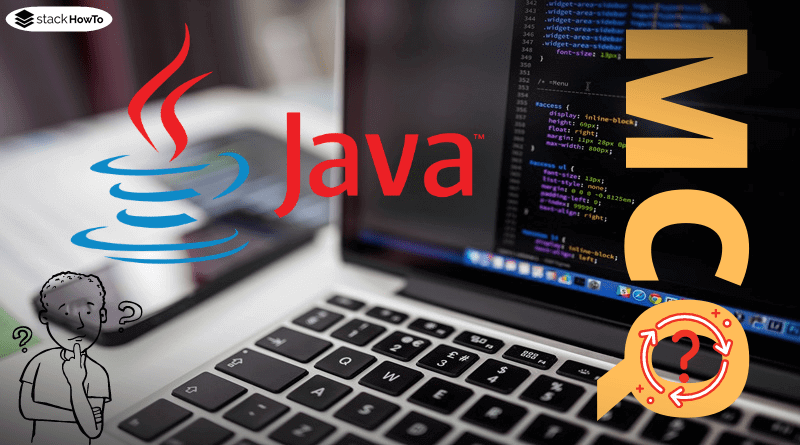java

# How to compare two objects in Java

When are two objects “equal”? This question always causes confusion. The key to understanding it is to learn the difference between the relational operator == and the equals() method inherited from Object. You will see: In the end, there is no longer any comparison.

###### Topic in short form
• The comparison operator == tests whether two reference variables point to the same object.
• The equals() method is used to compare objects according to their content. However, this must still be overwritten accordingly.

###### Identity of references

The comparison operator == has long been known when dealing with primitive data types. We can use it to test two primitive values for equality and get a Boolean value (true or false) as the result.

And what about objects? In principle, it is the same. Here is the rule:

The comparison operator == tests reference variables to see whether they refer to the same object.
Two references that point to an identical object on the heap are therefore the same. Here’s an example:

```Point p1 = new Point(2,2);
Point p2 = new Point(2,2);
Point p3 = p1;

System.out.println(p1 == p2); // false
System.out.println(p1 == p3); // true```

The two Point objects, to which the references p1 and p2 refer, are instantiated with the same parameter values. But this does not matter, because they are two different objects. Therefore the test p1 == p2 false.

The object p1 is assigned to the reference variable p3. This means that p1 and p3 refer to the same object. So p1 == p3 is true.

The comparison is based on the hash code of the objects. The hash code is a unique number that is uniquely assigned to each object. The method hashCode() inherited from the Object class can be used to prove the identity of each object (p1.hashCode() and p3.hashCode() return the same value):

```System.out.println(p1.hashCode());   // 931004833
System.out.println(p2.hashCode());   // 3634712458
System.out.println(p3.hashCode());   // 931004833```

Our code can be graphically illustrated in this way:Java MCQ – Multiple Choice Questions and Answers – OOPsThis collection of Java Multiple Choice Questions and Answers (MCQs): Quizzes & Practice Tests with Answer focuses on “Java OOPs”.   1. Which of the…Read More

###### The method equals()

With the equal-to operator (==) we can now check the identity of the objects. If we want to compare objects with each other, we have to look for another different way:

To compare the state or content of individual objects with each other, we use the method equals(), but we have to adapt it by overwriting it.

In the overwritten equals() method we then define how exactly the objects of a class should be compared. This means: We have to define ourselves when two objects are equal in content.

In our example above, the class (Point), this makes sense: Point objects are the same in terms of content if the instance variables have the same values.

So the overridden equals() method looks like this:

```@Override
public boolean equals(Object obj){
if(obj instanceof Point){
Point p = (Point) obj;
return (x == p.x) && (y == p.y);
}
else{
return false;
}
}```

The object obj passed in the parameter is compared with the object on which the method is called (in our case, the two Point objects p1 and p2 have the same content):

`System.out.println(p1.equals(p2)); // true`

Let’s briefly discuss the implementation of the overwritten equals() method:

Since the parameter of equals() must be of the type Object, we can send practically any type of object into the method. But logically only objects of the type Point shall be compared. Therefore, we first check in the method with the instanceof-operator whether obj is an instance of the class Point.

If the result of the type check is false, the method is immediately terminated with the return of false.

Before the comparison can start, obj must be cast to the reference type Point (currently the reference variable is still Object).

Only now are the instance variables of the two objects compared. If the values of the instance variables x and y are the same in both objects, the objects are considered to have the same content. The method is terminated with the return of true. Otherwise, the method ends with false (the objects are then not identical in content).

###### Question: Why overwrite equals()?

A good question! Why do we have to override equals()? If we take a look at the “original method” implemented in Object, we quickly see why overwriting is necessary:

```public boolean equals(Object obj) {
return (this == obj);
}```

By default, equals() only compares the references and not the objects. Comparing objects of a class is something that the compiler cannot possibly do automatically. How should it know how to compare the structure or content of our objects? So we need to tell him by overriding the equals() method in a meaningful way.Autoboxing and Autounboxing in JavaAutoboxing is the automatic conversion of a primitive data type into its wrapper class. Autounboxing refers to the automatic conversion of a wrapper class into…Read More

###### Compare Strings

When learning Java, one encounters primitive data types and strings. This leads many to compare strings like primitive data types with the == operator:

```String x = "Welcome to StackHowTo!";
String y = "Welcome to StackHowTo!";
System.out.println(x == y); //true```

In fact, in this example the result is true. But we can already see in the next example that strings cannot be compared with ==:

```String x = "Welcome to StackHowTo!";
String y = "Welcome to ".concat("StackHowTo!"); // add "StackHowTo!"

System.out.println(x == y); //false```

Although both strings “Welcome to StackHowTo!” the result is false. What is the explanation for this phenomenon?

Java stores a string literal only once in memory. In the first example, the two variables x and y point to the same object in memory. Therefore the result with == is true.

In the second example, x == y returns false, although both variables have the same string (“Welcome to StackHowTo!”). The reason is that we have two different String objects here. Because the string of y is only created at runtime by the method call. Since x and y are not the same when compiled, a new String object is created.

Therefore, the following law is absolutely mandatory for the string comparison:

• Never use == for the string comparison, but always equals().

It is practical that we do not have to overwrite equals() because the String class has already done this for us. So we can start right away:

```String x = "Welcome to StackHowTo!";
String y = "Welcome to ".concat("StackHowTo!"); // add "StackHowTo!"

System.out.println(x.equals(y)); // true```

So it looks good!Java 8 Stream TutorialOne of the big innovations of Java 8 is the new Streams API (Package java.util.stream). A stream in the sense of this API is simply…Read MoreMCQPractice competitive and technical Multiple Choice Questions and Answers (MCQs) with simple and logical explanations to prepare for tests and interviews.Read More